# Step-by-Step Math

December 1, 2009 —Comments Off

## July 17, 2019 Update: Step-by-step solutions has been updated! Learn more.

Have you ever given up working on a math problem because you couldn’t figure out the next step? Wolfram|Alpha can guide you step by step through the process of solving many mathematical problems, from solving a simple quadratic equation to taking the integral of a complex function.

When trying to find the roots of 3x2+x–7=4x, Wolfram|Alpha can break down the steps for you if you click the “Show steps” button in the Result pod.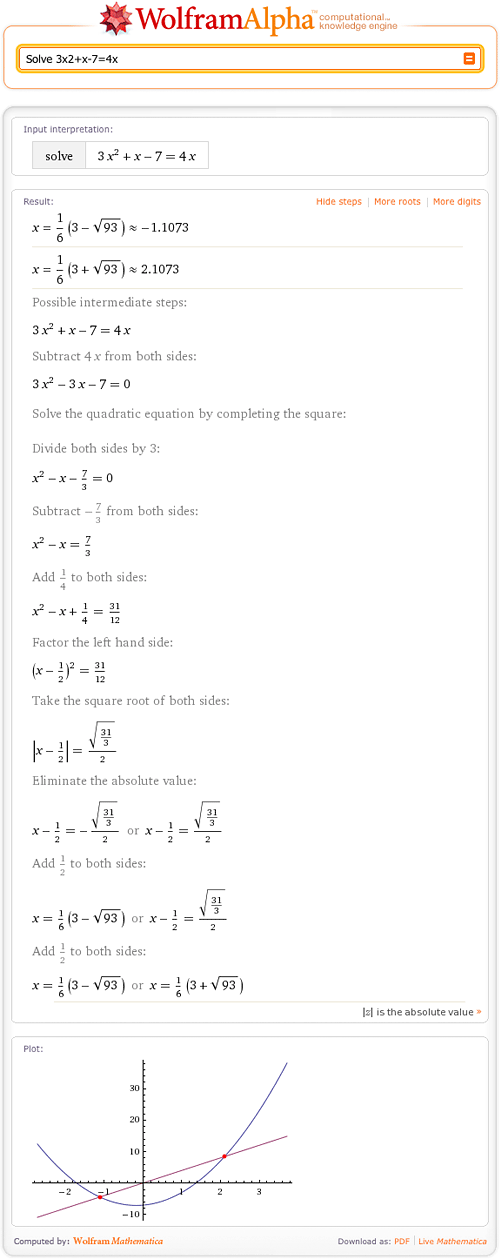As you can see, Wolfram|Alpha can find the roots of quadratic equations. Wolfram|Alpha shows how to solve this equation by completing the square and then solving for x. Of course, there are other ways to solve this problem!

Wolfram|Alpha can demonstrate step-by-step solutions over a wide range of problems. This functionality will be expanded to include steps for solutions in other mathematical areas. Look through the following examples to see the abilities of the “Show steps” functionality.

If you need to learn how to do long division of polynomials, Wolfram|Alpha can show you the steps. Let’s try (x5–14x4+3x2–2x+17)/(2x2x+1):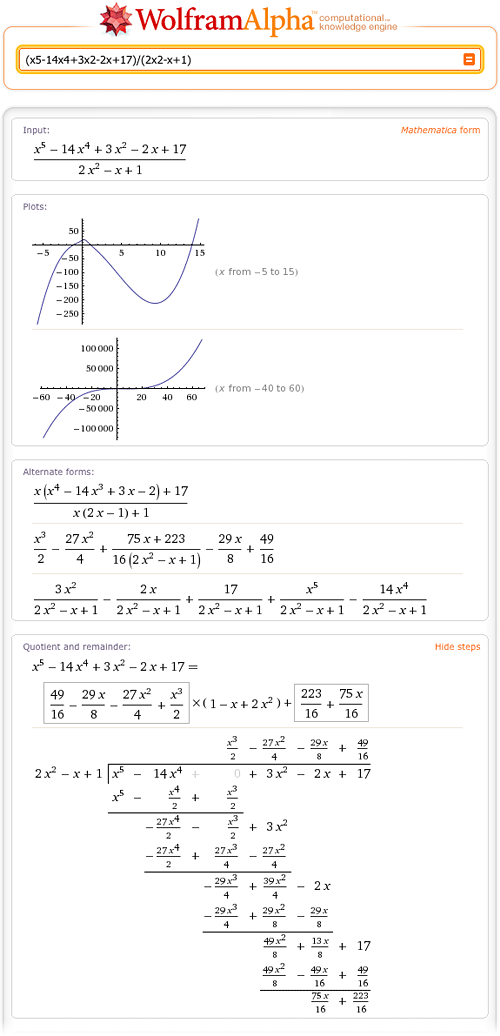If you are stumped trying to find the limit of xx as x->0, consult Wolfram|Alpha: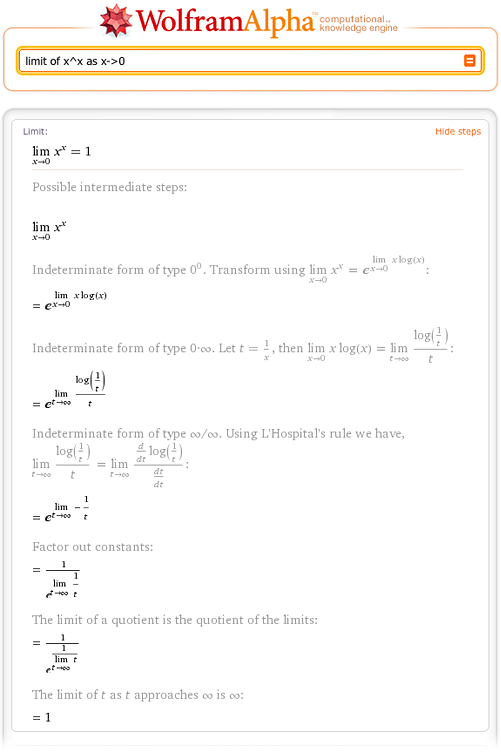When you need to find the derivative of (3x2+1)/(6x3+4x) for your calculus class, Wolfram|Alpha will find this derivative using the quotient rule.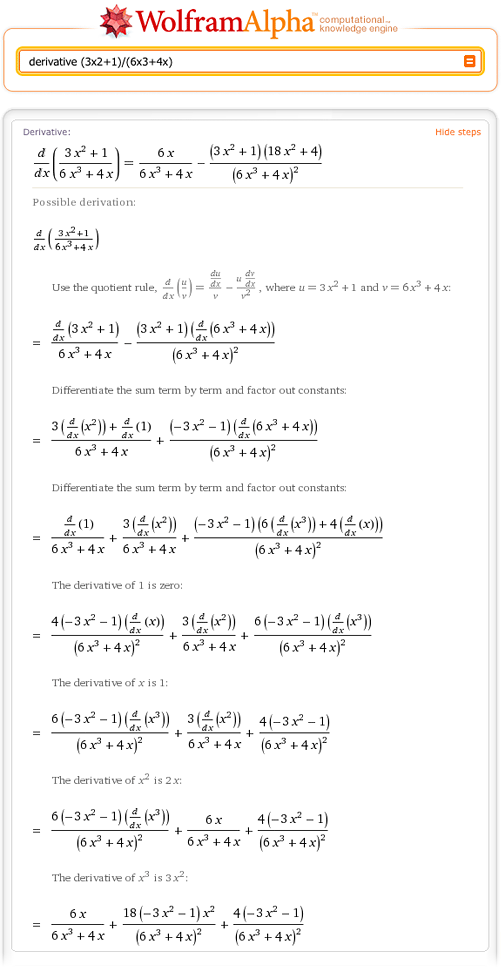Are you trying to integrate e2 x cos(3x), but forgot the formula for integration by parts? Wolfram|Alpha will remind you how to integrate by parts.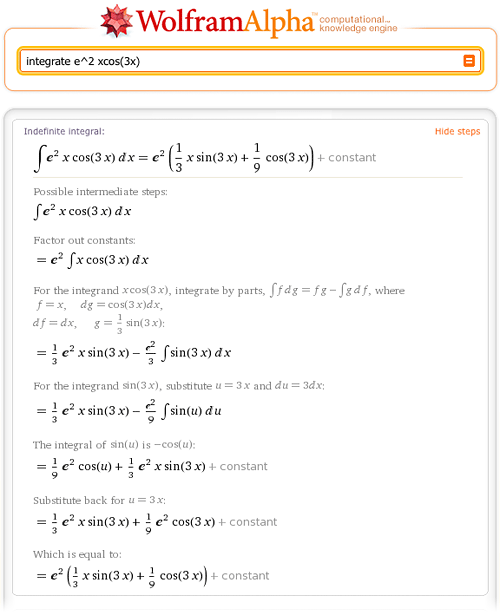Wolfram|Alpha can do virtually any integral that can be done by hand. Try the integral of x?[1–?[x]]: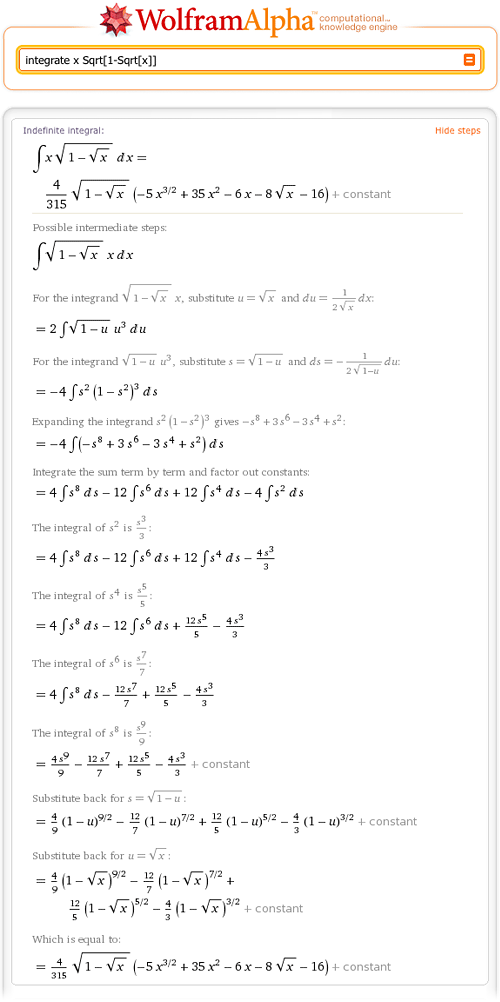Wolfram|Alpha also has the step-by-step functionality for partial fractions. Try partial fractions of 1/(x3–1):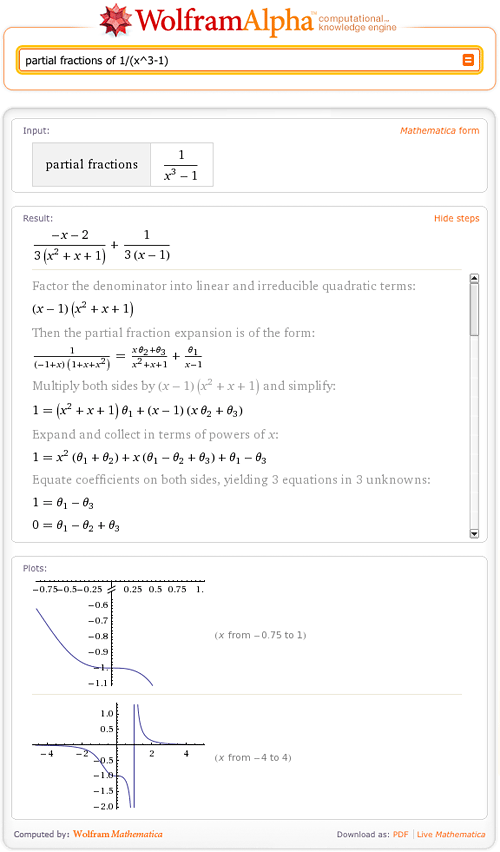The step-by-step programs in Wolfram|Alpha rely on a combination of basic algorithms and heuristics including Gaussian elimination, l’Hôpital’s rule, and Bernoulli’s algorithm for rational integration. These heuristics are a logical formulation of the natural methods used by humans for solving problems. By utilizing Mathematica’s powerful pattern-matching capabilities, Wolfram|Alpha’s developers have morphed these rules into a platform for breaking down and structuring the solutions to complicated problems, which closely mimics the ways by which a human would solve problems of these natures.

The “Show steps” feature allows you to learn basic mathematics on your own, or it can simply be a nice way to check your work! It can also give you insight on different ways to solve problems. So next time you find yourself ready to give up on a math problem, make sure to check with Wolfram|Alpha. Visit the Wolfram|Alpha Homework Day Gallery for examples of how you can use Wolfram|Alpha as a learning tool for other subjects.

Am I the only one to have noticed that the steps text is incorrect.
In text it say subtract 7/3 sides when in the equation its add 7/3 to both sides.

Posted by Adam Speight December 2, 2009 at 7:29 am

It says subtract -7/3, which is the same as adding 7/3.

Posted by Not Adam Speight December 2, 2009 at 3:28 pm

No, it’s just clunky. It says subtract -7/3 i.e. add 7/3.

Posted by Adam K. December 2, 2009 at 5:15 pm

The text meant complete the square.

Posted by Adam K April 9, 2010 at 2:42 pm

That is so crazy.

Posted by tyler March 30, 2014 at 3:40 pm

Thankyou for pointing out how the ‘Show Steps’ function is triggered. I had heard of it but never met it in use. There are many such links on the answers which are easily overlooked.

Posted by Brian Gilbert December 2, 2009 at 8:45 am

I would like to know how to plot a grayscale with Wolfram Alpha. I apologize if this comment is off site.

Posted by Miguel December 2, 2009 at 8:56 am

Miguel, i’d like also to know it. Sorry for offtop, nut explain us please 😉

Posted by sexvkontakte December 2, 2009 at 10:03 am

This is awesome!!!, but was it really worth the work :/ ???

Posted by David Mulder December 2, 2009 at 1:00 pm

This is pretty nice… I would like to see the interface enhanced so I could enter several math problems at once (for example: Chapter 7, problems 1-19 (odd)) and have the output in a printable / customizable format (ie a script font that looks like my handwriting).

But, this is definitely a great start! Thanks 🙂

Posted by HIGH Schooler December 2, 2009 at 3:06 pm

I lol’d.

great idea brah

Posted by Another HIGH Schooler December 2, 2009 at 4:05 pm

That would be really sweet, but that would require going through all the textbooks in all the high schools of the country and it recognizing where you are to base your school district and analyzing the textbook that that district uses.

In other words, it’s impossible.

Love the idea though.

Posted by BlockJuice December 4, 2009 at 7:52 pm

How many people thought Wolfram Alpha would be possible 10 years ago…

Posted by college September 22, 2010 at 6:59 pm

Wonderful, this looks fantastic, great work. I can’t belief it!

Posted by marasan December 2, 2009 at 3:18 pm

Love this. Where is it going?

Posted by SusanZR December 2, 2009 at 4:15 pm

Wolfram|Alpha rocks my socks.

Posted by rudolpho December 2, 2009 at 4:17 pm

I use W|A with my Grade 9 students as we solve linear equations and go over the Show Steps button. They were amused because it is inefficient: it equates to zero first and then isolates the variable. (Please keep it that way…)

Posted by Cal December 2, 2009 at 4:35 pm

I wish this had existed when I was still in school. Easily one of the most exciting things I have seen recently.

Posted by Andres Barreto December 2, 2009 at 5:05 pm

Wolfram|Alpha. Hacks for school.

Posted by BlockJuice December 2, 2009 at 7:22 pm

When are we going to be able to define some variables of a function as constants and not variables??! This is tremendously limiting.

Posted by Dude December 2, 2009 at 8:09 pm

congratulations on killing textbooks, wolfram. I’m sure scores of old people will be telling kids about their hey-day now.

Posted by mitch December 2, 2009 at 10:55 pm

absolutely stunning!

Posted by balaji December 3, 2009 at 12:26 am

wow now it is going to help me a lot… because I am very poor in maths.
Thanks

Posted by dhiraj December 3, 2009 at 1:10 am

Wow, really really impressive. Great work guys!

Posted by Edward December 3, 2009 at 3:18 am

Sounds cool! great!

Posted by Daniele Simonin December 3, 2009 at 6:37 am

show that

3^2008 + 4 ^2009 can be written as product of two +ve integers each of which is larger than 2009 ^182

Posted by A Mukherjee December 3, 2009 at 7:18 am

This is mostly curiosity, but why is there now a post about this feature? Hasn’t it been implemented since wolfram alpha first started?

Or has there been a major overhaul that shows full working/solutions for more types of equations? The equations I tend to use it for, usually says (step-by-step results unavailable’

anyway this feature is awesome, and I use it quite a lot to aid my learning.

Posted by chris December 3, 2009 at 7:36 am

Awesome! I wish I had this feature few years ago while studying at University.
I was solving a lot of math helping other students and raising my wellfare, and this feature would be dramatically useful.

Posted by digi December 3, 2009 at 8:11 am

Oww.. Very curious. Awesome!

Posted by Marcelo Borth December 3, 2009 at 10:58 am

They are all easy except the partial fraction which is kind of different. Not hard but different lol lol

Posted by Master d Or December 3, 2009 at 2:44 pm

How to write 0.5 (1/2) in wolphram as logarithm base?

Posted by guest December 3, 2009 at 3:54 pm

The log to the base a of b is the same as
log(a)/log(b)

Posted by Bill Kamp December 8, 2009 at 3:21 pm

Hi

To obtain the logarithm with a fraction as base you have to write the base without the 0 before the decimal point:
Log .5 of (x^2+2x)
To obtain a logarithm in base 1/2

Hope it helps, it sure took me a while 🙂
Greets and thanks to the people behind WA!

Posted by Andrei April 13, 2012 at 1:31 pm

Better yet, use the Wolfram Mathematica notation:
Log[base,ecuation]

ex: log[1/2,x^2+x]

it is always a good ide to look for the mathematica syntax

Posted by Andrei April 13, 2012 at 1:55 pm

I think I’m in love :O

Posted by vampirecow December 3, 2009 at 6:42 pm

If you try to solve a limit, but the left-handed limit and the right-handed limit are not the same, Wolfram Alpha gives both one-sided limits, but the “show steps” button doesn’t appear. Is there a way to make it come up? Or to request only one of the one-sided limits and have the button appear?

Posted by Jon December 3, 2009 at 10:32 pm

f(x) = x^2 – x + 1, f((2+h) – f(2))/h.

How could I input this equation to get a step by step process to solve this difference quotient?
Tried it. But wolfram simply spit back the equation back at me, not the solution.

WS

Posted by will December 4, 2009 at 11:20 pm

a good site but it must also open for other branch of math

Posted by aksh December 5, 2009 at 2:51 am

this is totally fantastic and very useful to all students.. this is the very beginning of great change in terms of search engine ..

Posted by duncan thorne December 7, 2009 at 1:14 am

very beautiful site it is awesome it is a good tool for all students .it will be very nice if they can include more fields of mathematics.

Posted by sami December 27, 2009 at 2:20 am

The “show steps” botton does not show in any of the problems I had to do 🙁 I’m sad.

Posted by lydia January 13, 2010 at 1:16 am

The computations this can do are very impressive, especially with integration.
However, i wish there was a way to modify the input to perform integrals according to specific methods i.e. simpson’s rule, partial fractions, ect.

Does anyone know if this as the ability, or does it have to be done specifically in a notebook?

Posted by Cuyler Pittan February 14, 2010 at 10:54 pm

That’d be really useful!!! I think it’d be really cool if we could save problems that we’ve done, if need be. (There are times when I have to re-type my question because I’ve moved on and then decided to take another look at it.) Also, I wish W/A could compute answer for the Trapezoidal Rule without a condition. (None of my math problems for Trapezoidal Rule ever have conditions. They only ever give me the equation and say “with n = 8”.

Posted by Stephanie December 12, 2013 at 3:27 pm

This is very beautiful site for the children and it will help lot more to others who are not understand the maths properly.

Posted by Optimum Reading February 19, 2010 at 6:24 am

Can you solve this;
Ten not necessarily distinct positive integers have the property that if all but one are added, the positive results are 82, 83, 84, 85, 87, 89, 90, and 92.

I need some help with this.

David

Posted by David Sherrod March 2, 2010 at 3:43 pm

I notice that maths solving problem is not correct. If any one can solve this properly.

Posted by Hassy March 5, 2010 at 12:38 am

No one can answer my question how it could be solve

Posted by Hassy March 15, 2010 at 4:56 am

Nice awesome. really cool one.

Posted by Alok Kumar March 24, 2010 at 8:05 am

Really awesome, many thanks for this !!

Posted by Sauce Tomate April 1, 2010 at 4:58 pm

Absolutely right ! It is great work when you did All the possible steps while solving the mathematical problems you have to keep in mind that every step is necessary if you want your answer correct.

Posted by Alison J. Castro September 24, 2014 at 7:55 am

Guys is incredible what you has achieved lately!

btw the link of the limit x^x example is wrong:

http://www.wolframalpha.com/input/?i=limit+of+xx+as+x-%3E0

it should be:

http://www.wolframalpha.com/input/?i=limit+of+x^x+as+x-%3E0

Posted by Daniel "warmth" Delgado April 8, 2010 at 3:41 pm

Thank you, Daniel! We’ve fixed the link.

Posted by The PR Team April 8, 2010 at 9:24 pm

Math is not for for me but still i have to study it 🙁 😀 great work though

Posted by Rohit April 14, 2010 at 6:55 am

It is not showing steps for negative exponents.

like: (2x^0Y^2)^-3 * 2yx^3 Wow thanks for the answer but how did you do it?

Posted by mls May 22, 2010 at 5:00 pm

It is not showing steps for negative exponents.

like: (2x^0Y^2)^-3 * 2yx^3 Wow thanks for the answer but how did you do it?

Posted by A?k Büyüsü Nas?l Yap?l?r June 5, 2010 at 8:10 am

Can you enter negative exponents?? Have not been able to do it.

Posted by Mo June 12, 2010 at 10:48 am

What a informative post Step-by-Step Math in wolfram alpha blog. Really problems steps discussed in detail manner. I like this. I show this post to everyone in my family. Really i enjoyed most. Thanks for sharing a very informative blog.

french

Posted by Susan July 30, 2010 at 3:19 am

Wow, I’ve got to get deeper with my maths skills. It looks neat but I got lost pretty quickly:)).

Posted by Pokrycia Dachowe August 9, 2010 at 10:37 am

What an amazing search engine. I don’t know what to say thay you guys at Wolfram|Alpha really did a greeeeeaaat job making this such amazing engine into operation. I just wonder how math student use this engine on their exams. Should they solve the equation manually, or just put it inti Wolfram|Alpha?

Posted by Alex Partoba August 13, 2010 at 6:04 am

WOW. I have never seen anything quite like it before. Solving maths problems in a search engine that even show steps and people thought google was the best, not anymore. I tried tanx/x without limits and as x approaches 0 and result BING. I am just so impressed, mathematics happen to be my favorite subject and i think i am going to spend my free time here. Next i tried TNT, it gives options as a finance entity, chemical compound so i choose chemical compound and i get the forumula, structure, IUPAC name and properties. This will take education to a new level.

Just want to ask are those values fed in database or calculated in real time and then cached.

Will be back soon.

Posted by funny September 4, 2010 at 5:05 pm

its very interesting very helpful site 2 those who r really interested in math

Posted by chetana.toppalad September 11, 2010 at 5:11 am

so, i used wolfram to find x in 4^x-3^(2x)=6 but i cant seem to find the steps. any help?

Posted by heya September 12, 2010 at 11:10 am

this is totally fantastic and very useful to all students.. this is the very beginning of great change in terms of search engine ..

Posted by michaelosimms.com October 6, 2010 at 8:19 am

What a informative post Step-by-Step Math in wolfram alpha blog. Really problems steps discussed in detail manner. I like this. I show this post to everyone in my family. Really i enjoyed most. Thanks for sharing a very informative blog…thanks a lot………….

Posted by city macash e October 13, 2010 at 1:23 am

Hey
Doing some math and using Alpha to check my answers, its fine when they are correct but when they aren’t I cant check the correct calculations like you show here in this step by step post. I have no show/hide steps button. Any suggestions?

Posted by Andre October 14, 2010 at 6:52 am

Hi Andre, Can you provide us with a few of the inputs that you tried? We’re happy to take a look.

Posted by The Wolfram|Alpha Team October 14, 2010 at 8:45 am

integrate 2 sqrt(1-(x^2)/9) from x=0 to 3

Posted by Maria October 26, 2010 at 1:27 am

Hi, I have a similar problem with one limit:
http://www.wolframalpha.com/input/?i=lim_%28x-%3E0%29%28sin%28x%29%2Fx%29%5E%281%2Fx%5E2%29
The answer is correct but when I want to see steps it says “step by step result unavailable”.

Posted by Fanda October 27, 2010 at 5:21 pm

pretty much any derivative i try to calculate it gives me the answer but i cant see the steps. for example, derivative of ((sin x)^-5)-((cos x)^3). i used wolfram alpha to solve this and many other problems a few days ago and it showed the steps just fine but now it won’t. wondering what’s going on thanks

Posted by Ally November 3, 2010 at 3:40 pm

it won’t show work for any of the problems i put in

Posted by Gigi December 1, 2010 at 11:51 pm

If only, i came across this article during my school days……..thankfully, i am off maths. anyway great post for all the folks that are struggling with maths.

Posted by magic of history October 28, 2010 at 5:15 am

i just tried doing the derivative shown as an example in this post and and not even that one would allow me to see the steps. very frustrating!

Posted by Ally November 3, 2010 at 3:43 pm

Hi Ally,

Can you give us the an input that you tried. We will check it out. Thank you.

Posted by The Wolfram|Alpha Team November 4, 2010 at 3:43 pm

The show steps isn’t working for any derivatives I’ve been trying. 🙁

Posted by Steve November 5, 2010 at 2:00 am

Hi Steve, We’re happy to look into this. Can you give us the input that you tried? Thank you.

Posted by The Wolfram|Alpha Team November 5, 2010 at 7:37 am

By the name of Allah the most merciful and the most gracious

the wolframeAlfra site is fantastic and very good website

I will tell all my friends about it

Thanks for this good work

Posted by Ahmed November 4, 2010 at 12:38 pm

When I enter y”+4y=(x^2-3)sin(2x), I cannot see the steps. this would be helpful because I also did it by hand and I got a different answer (the one given from Wolfram is correct). It would be amazing to figure out where I made my mistake

Posted by Nate November 5, 2010 at 5:06 pm

No matter what function I put in, it seems as though the show steps part of the derivative doesn’t work. I click show steps and it looks the same as it was before.

Posted by nick November 6, 2010 at 11:41 pm

Yeah, the show steps for derivatives does not seem to be working. At least it isn’t working for me.

Posted by Mike November 7, 2010 at 12:07 pm

I have been using the “show steps” and it was working perfectly until a few days ago. I too have been trying to take derivatives and the answers are correct, but it will not display the steps (I also have the iPod app and the same problem is happening there. An example I’ve tried is: y = (x-3)/(4x+2)

Posted by Jane November 7, 2010 at 5:34 pm

I haven’t been able to get any of the “show steps” to work for derivatives. I have tried using different browsers (Chrome, Firefox, and IE7) tried adding and removing add blocking software. Nothing seems to have any effect whatsoever, when you click “show steps” the web page loads like it’s attempting to show the normal step-by-step but doesn’t ever display anything, even though the button does change to “hide steps” no other changes are visible. Please fix this website, to me this is the alpha and the omega (pun intended), and I can’t wait to have full functionality back!

Posted by Chris November 8, 2010 at 11:52 pm

Hi all, Thank you for bringing this to our attention! Our team is working to get this back up and running. Thank you.

Posted by The Wolfram|Alpha Team November 8, 2010 at 11:56 pm

I have also purchased the Wolfram Alpha mobile application (Android OS) and this same show steps functionality is as it is on the rest of the site, not working.

Posted by Chris November 8, 2010 at 11:58 pm

It would be awesome to have the steps on
FullSimplify[(2 – Sqrt[2 + Sqrt])/Sqrt[2 – Sqrt]]

(I am using a slow connection. I heard this might be the problem?)

Posted by Raptortech97 November 9, 2010 at 1:19 pm

No, it doesn’t work.

Posted by _blindsniper_ February 17, 2011 at 1:36 pm

If my radius=1 ,what would be the height of the line from the tangent point if it covers 25 percent of the circle area.

Posted by Peter November 10, 2010 at 1:29 pm

Although I see that for some maths the site is now showing the steps again I cannot get the show steps for 2nd order ODE’s such as:
2y” +y’ +3y = x^2 +6

What would I need to type in to get the steps, or do they simply not show for this type of maths, or is the maths still not 100% working!

Posted by Charlotte November 22, 2010 at 5:37 am

lim to infinity (n)/(n!)^1/n doesn’t work either

Posted by Manuel November 25, 2010 at 6:01 pm

Whenever I input a problem with i (the imaginary number, sq.root of negative one) it doesn’t show the solve button :s

Posted by Zeff November 29, 2010 at 10:19 pm

whenever i type in an equation ill either get a “result” (which im assuming is the answer) or i just get nothing at all (which is most of the time) how come im not getting any answers?

Posted by amanda December 9, 2010 at 11:00 pm

Hi Amanda,

Can you provide us with a few of the inputs that you tried? We’re happy to take a look. Thank you!

Posted by The Wolfram|Alpha Team December 10, 2010 at 7:05 am

I just wanted to say, i’ve been with Wolfram alpha since the day it went public back in may 2009, and i have to say this site has helped me more though my advanced math classes than any calculator, textbook or teacher ever could. thanks for making this site what it is!.

Posted by Jason December 22, 2010 at 12:57 am

When I enter integrate (3^x)/(4-9^x)dx, I cannot see the steps. this would be helpful because I also did it by hand and I got a different answer (the one given from Wolfram is correct). It would be amazing to figure out where I made my mistake

Posted by Serg January 13, 2011 at 12:06 pm

Hello Serg, Wolfram|Alpha doesn’t show steps for every function yet. We are continually working to support more step-by-step functionality. Thank you!

Posted by The Wolfram|Alpha Team January 16, 2011 at 11:23 pm
Posted by Serg January 13, 2011 at 12:12 pm
Posted by Caterpillar January 30, 2011 at 12:41 pm

ITS not working again
factor 500a^3+32

Posted by zac February 12, 2011 at 4:14 pm

Jumping on the bandwagon here: the show steps feature doesn’t seem to be operational for this problem:

integral of (cos(n * cos^-1(x))) from -1 to 1

Posted by shonte February 14, 2011 at 8:41 pm

when i type factor 500a^3+32
it does not show the steps

Posted by zac February 15, 2011 at 5:53 pm

how to solve limit r(x)/(1+x2)

Posted by philip March 8, 2011 at 10:38 pm

Hi, i have done 20 diferent questions online and NONE have come up with a more steps option. Example : y-2=((-5-2)/(-3+1))(x+1)

Posted by Danielle March 19, 2011 at 2:05 am

It does not work when you enter an definite integral :/

Posted by Reuben March 23, 2011 at 4:17 pm

Posted by Matt March 28, 2011 at 5:23 pm

Hello Matt,

We don’t support step-by-step for LaPlace Transforms yet either… we will in the future.

Thanks!

Posted by The Wolfram|Alpha Team April 1, 2011 at 10:10 am

When will step-by-step support for LaPlace transforms be implemented (at least providing table used)?

Posted by Tom July 7, 2012 at 8:18 pm

I cant seem to get steps for

integrate (1/(x(pi-2*x))) [(pi/6),(pi/3)]

Posted by Tharkaan March 29, 2011 at 3:16 am

Hello Tharkaan,
Our developers are continually adding step-by-step support for math problems. At this time, this specific integral is not yet supported.

Posted by The Wolfram|Alpha Team March 29, 2011 at 2:57 pm

I can’t get steps to show for anything. I even tried using the identical limit example pictured above and the steps don’t show for me.

Posted by abney317 March 31, 2011 at 9:16 am

Hi abney317,

Thank you for bringing this to our attention. Would you mind telling us what browser/operating system you are on?

Thank you,
Wolfram|Alpha Team

Posted by The Wolfram|Alpha Team March 31, 2011 at 2:37 pm

Oddly enough it works fine at my home computer.
At school I was running FF 3.6 on Win7… didn’t work on IE 8 either…

at home I’ve got FF 4 on Win7 and it’s working perfectly

Posted by abney317 March 31, 2011 at 9:56 pm

Very useful stuff. Even a novice can understand easily.

Posted by Education transcription June 10, 2014 at 2:43 am

Hi,
I need to solve summation problems. but I cannot get step by step solution for summation problems. do you provide step by step solution for summation problem or not???

Posted by nini March 31, 2011 at 8:25 pm

http://www.wolframalpha.com/input/?i=integrate+1%2F%28x^2%2B4*x%2B5%29+from+x%3D-2+to+-1
steps do not work

Posted by tana April 1, 2011 at 5:07 am

Hello Tana,

We don’t support step-by-step for this yet… we are constantly adding new step by step functions everyday. Stay Tuned!

Thanks!

Posted by The Wolfram|Alpha Team April 1, 2011 at 10:18 am

I’ll pay for the program that shows step by step on a consistent manner. Wolfram Alpha is fantastic and don’t get me wrong Mathematica is also amazing, but I can’t believe this key area for students hasn’t been more developed. It would be a sellout instantly. Please help! Thanks.

Posted by Mike April 11, 2011 at 12:22 am

Then check Mathway.

Anyway, I tried some inequalities and the examples pictured in the above blog post, but step by step didn’t show up.
I’m on chrome (win7).

Posted by kache May 25, 2011 at 8:21 am

Is there somewhere where we can check what functions have full or partial “step-by-step” functionality? Would be great to have.

And is there any step-by-step functionality in the algebra section? I’d really like to show WA to my students, but the course is for algebra, and it’s not very good if they can only get the answers without the full explanation.

Posted by Art April 16, 2011 at 6:03 pm

It was awesome step by step. thank you

Posted by saeid April 27, 2011 at 7:51 am

dane jest równanie okregu x2+y2-2x-3=0
zapisz równanie tego okregu w postaci kanonicznej i wypisz wspó??edne oraz promie? okr?gu

Posted by papitek310 May 5, 2011 at 1:46 pm

A C.N.A. offeres a patient 10 ounces of water. The patient drinks 180 milliliters of water. How much is left?

Posted by Staci May 5, 2011 at 6:53 pm

integrate [x/(x^2-2)^4] from – infinity to -4

No show steps!

Posted by mike May 12, 2011 at 3:36 pm

when i try to work out the simultaneous equation y=2x-7 and x^2+y^2=61 it wont show steps is this because it works it out using a graph if not then how can i see the steps?

Posted by Tom May 18, 2011 at 10:56 am

hi..I would step by step in operation with matrix, lowreduce, solve ecc ecc..is possible? thk!!

Posted by francesco May 29, 2011 at 3:42 pm

Hi

I haven’t been able to get any of the “show steps” to work for definite integrate.

I just want to know if you don’t support step-by-step for this yet.

Thanks

Posted by Nestor June 1, 2011 at 5:54 am

As far as i’ve seen you can only show steps for functions, etc.
Why is there no ‘show steps’ button for normal calculations (not involving variables) such as
4 / 2^(3/2) ? For lower-level mathematicians like me, who don’t understand the steps going from 4 / 2^(3/2) to sqrt(2) this is very difficult to find out.

Posted by Sam June 4, 2011 at 10:14 am

[…] show one way of reaching the solution with the “Show Steps” button. Check out the post “Step-by-Step Math” for more on this […]

Posted by Wolfram|Alpha Blog : More Math Help from Wolfram|Alpha June 7, 2011 at 10:42 am

[…] in a wide variety of areas, including finance, nutrition, chemistry, astronomy, math, travel, and even solving crossword […]

i want to calculate complex varible. step by step. so that program don’t show step on

Posted by gargata June 16, 2011 at 10:00 am

I tried putting in an equation, and the answer came up fine, but no show steps button is to be found :/

Posted by Wagner June 27, 2011 at 8:20 pm

please I need a step by step answer to my question,, My question is:
16 ounces of fresh juice contains 216 calories and 16 ounces of grapefruit juice contains 174 calories. If 8 ounce mixture of these 2 juices contains 94 calories then what fraction of the mixture is the orange juice?

Posted by ahmad July 10, 2011 at 8:36 am

I am looking for some more basic algebra skills etc for Middle school math. I am not finding step by step math for this age group. Please advise… Is there a place I can find it? Or a way to add some examples?

Posted by Rachaael Curry July 13, 2011 at 10:33 am

Does Mathematica have this “show steps” capability?

Posted by Nick July 21, 2011 at 4:12 pm

Wolfram|Alpha Mathematica used to be the source I would go to in order to check my work and answers. Unfortunately, now that I need it again for my Calculus 3 course, the answer will come up, however the “step-by-step” option is no longer there. The most important part is making sure I know how to do the problems correctly, not just the answer. Has this option permanently been discontinued? If not, when will it be back?

Posted by Knarf July 31, 2011 at 10:52 pm

I want to know too.

Posted by jon August 29, 2011 at 5:29 pm

Find a factor (divisor) of
12702047 other than 1 & itself

Posted by shaheer August 4, 2011 at 7:50 pm

Hey Wolfram ALpha – I have here a simple sqrt and I want to know the solution to this:
((2*sqrt(3))/sqrt(2))^3 – it shows me the solution but I dont know how you solved this.

Posted by Wie August 9, 2011 at 5:37 am

How do I input a table of paired values to get a fitted curve?

Posted by Raymond L Kornele August 30, 2011 at 8:54 pm

There are some diiferential equations for which wolfram alpha doesn’t give me the show steps options. Why is that. The equation i put in was this; y”[x] + 3*y'[x]*y[x] + (y[x])^3 = 0. And this y”[x] + x/2 y[x] = 0. The second one I can understand as it’s a special function but it should give the option for the first one at least.

Posted by Nihav September 13, 2011 at 8:53 am

Putting in -x = -8 won’t give me a show steps button. Neither will 2/9 + x = 1/3.

Posted by Troika September 13, 2011 at 6:12 pm

There are some expressions where it is not useful as it is supposed to be. For example with (d/dx a^x) it doesn’t show useful steps. For example, it could show the chain rule method.

Posted by SaduJ September 21, 2011 at 8:07 pm

Where do I find the “show steps” button?… It is not in the same place as on the illustrations

Posted by JOhan holm September 25, 2011 at 4:46 am

write the equation of the lie satisfying the givenn conditios. Label two points o the line.
parallel to y=4x+1 and passing through the point (0,-3)

Posted by Dave Kendall October 8, 2011 at 5:33 pm

Limit of {(2x-3)(sqrt x-1)}/(2x^2+x-3) as x approaches 1
please solve this and mail the solution to gbaidco@gmail.com.

Posted by anmol October 18, 2011 at 9:55 am

ln(sin(x))+12*x*sinx+1=0

Posted by maryam November 2, 2011 at 1:54 am

logp -log(p -2)=log5

Posted by OlaOluwa Familoni November 7, 2011 at 10:55 am

[…] Step-by-step math. […]

How do I print the step by step results? I get nothing in my print preview.

Posted by Lynn28 November 8, 2011 at 10:49 am

please show me solving step by step sum(1/j^2)j=1..infinity

Posted by omid November 12, 2011 at 1:46 am

when i enter:
solve x=sqrt(2)*sqrt((6-x)/x)

it gives me the solution but when i click on show steps, it tells me they are unavailable

Posted by venomzx November 28, 2011 at 10:46 pm

pls i need to solv this
1000000=x-(10%of x),what is x?

Posted by zainab November 30, 2011 at 10:03 am

Your program shows the answer to sum_(k=1)^(2000)2/(4^(k/2001)+2) but it doesn’t show how it has reached the answer.

Posted by Shahriar December 5, 2011 at 9:12 am

can you show step by step input to solve this integral using wolfram mathematica software?
integrate sin^n xdx for n = 1, 2, 3, . . . , 10.

Posted by nai December 14, 2011 at 11:30 pm

3x-3=-2x+2
andd :

Posted by haley December 19, 2011 at 8:05 pm

how do I use wolfram alpha if i want to find the second derivative ?

Posted by sylivia December 31, 2011 at 12:12 pm

Hello

Why does W|A answer “Step-by-step results unavailable” when I ask for the limit of sin(x) / x as x -> infinity ?

Thx

Posted by Greg January 2, 2012 at 4:51 am

This is a great tool to use when in need of math help 🙂

Posted by Amber :) January 2, 2012 at 6:29 pm

in simple example like 2/9 + x = 1/3 or 3x^2+x–7=4x, some step unnecessary. but this is great tool, it’s free, amazing…

Posted by dalee8x February 4, 2012 at 12:24 am

the function would be great — but I’ve found that in questions where there are separate tabs for “result” and other representations( i.e. derivative or indefinite) … the “show steps” only works for the representations. Neither of those alternate representations help me comprehend or answer the problem.

Posted by Griffin March 11, 2012 at 11:45 am

it should also be noted that “verification” — the process of showing two equations are equal to one another — simply states whether it is true or false, I have not seen an option to show the steps for the process it used.

Posted by Griffin March 11, 2012 at 11:49 am

y + (1/x) = 7/2,x + (1/y) = 7/3
NOR
partial fractions of [y + (1/x) = 7/2,x + (1/y) = 7/3]

Does not show steps

Posted by Trav April 20, 2012 at 7:34 am

1/(cosx^5sinx^3)^(1/4)

Posted by Pussican May 11, 2012 at 9:09 am

1/(cosx^5sinx^3)^(1/4) dx

Posted by Pussican May 11, 2012 at 9:10 am

PV=FV/(1 + i) N
PV=850/ (1+0.12)10
=850/3.10585 ( This is the step I do not understand how did we get 3.10585)?
=273.68

Posted by Avor Cooley November 29, 2012 at 2:52 pm

Posted by gabriella January 15, 2013 at 5:00 am

Very good step by step explanation.

Posted by Prathap June 2, 2013 at 5:44 am

Excellent explanation and information on solving the problem.

Posted by Education Transcription June 3, 2013 at 8:04 am

It’s really nice Post, I like the way of presentation..
thanks for such nice Post..

Posted by Jitendra Shukla July 10, 2013 at 5:01 am

I would like to know how to plot a grayscale with Wolfram Alpha. I apologize if this comment is off site.

Posted by acompanhantes sao paulo August 9, 2013 at 1:37 pm

Hello Serg, Wolfram|Alpha doesn’t show steps for every function yet. We are continually working to support more step-by-step functionality. Thank you!

Posted by acompanhantes sp sao paulo August 10, 2013 at 8:15 pm

Too bad that Wolfram Alpha doesn’t allow the step-by-step solutions for inverse of functions…:(

Posted by Jonathan August 19, 2013 at 6:27 pm

Really nice blog this will definetely going to help my kids to study extra. Thank you

Posted by Orice November 10, 2013 at 12:47 pm

useful mathematical Step-by-step solutions. good stuff…..

Posted by Zuan Education December 7, 2013 at 1:35 am

I don’t understand that question my self.

Posted by tyler March 30, 2014 at 3:44 pm

Great job! Is there an API for this? I know it’s part of the pro-subscription, so I’m guessing it’s “pay per query”.

Posted by Adam April 16, 2014 at 9:04 am

limit ((a*x+b)/(a*x+c))^x
x->+infinit

Posted by Vitor April 26, 2014 at 5:37 pm

A-B= Null set, if and only if,
i) A is proper subset of B
ii) B is proper subset of A
iii) A cap B = null set

give reason .

Posted by somnath June 18, 2014 at 6:14 am

When trying to find the roots of 3×2+x–7=4x? I not have idea of how make!!! 🙁

Posted by acompanhantes são paulo July 7, 2014 at 1:35 pm

Simplify :
2/3(1/3*8(2/5 of 15/18 – (3 – 5/2)))

Posted by Debasish Bhattacharjee August 4, 2014 at 2:45 pm

Simplify :
2/3(1/3*8(2/5 of 15/18 – (3 – 5/2)))

Posted by Filmes Online HD August 12, 2014 at 12:22 am

Como faço para ver a resolução passo a passo? quando carrego na opçao “step by step solutions” aparece-me “possible intermediate steps” mas nao me aparece os passos. Como hei-de fazer para os passos aparecerem?

Posted by Débora Ferreira September 2, 2014 at 6:01 am

speed in km/hr of earth moving around the sun, assume it is a circular path of radius 150 million kms

Posted by monique September 8, 2014 at 8:21 pm

How come when I push the show step button sometimes, it only give me a line written possible intermediate steps?

Posted by leung October 28, 2014 at 10:17 am

Actually not a comment. How can you find the inverse laplace transform of lns+1/s+4

Posted by James kolubah October 29, 2014 at 5:05 pm

Wonderfull.

Posted by M. KOUAME February 11, 2015 at 11:30 am

It ws fabulous! Yes indeed, it really helps me in some area that av lost doing my calculation

Posted by Hakym February 20, 2015 at 11:44 am

The step by step breakdown has helped so much, wanted to say thanks for wolfram|aplha and especially thank you for having an API!

Posted by step maths September 29, 2015 at 8:33 am